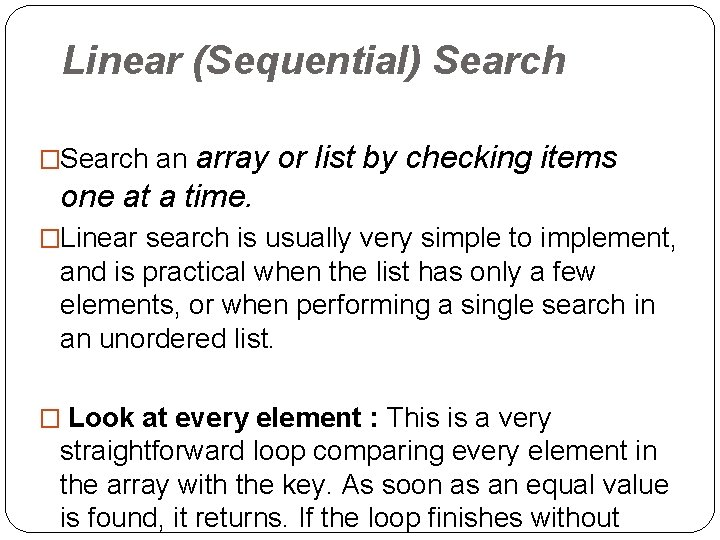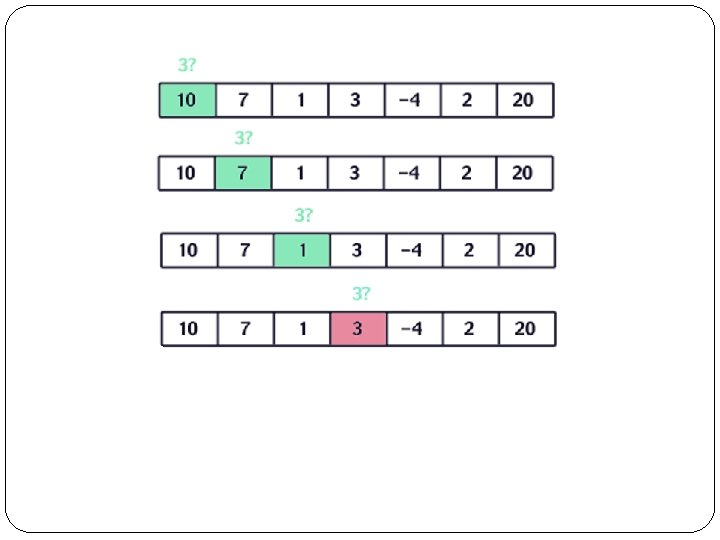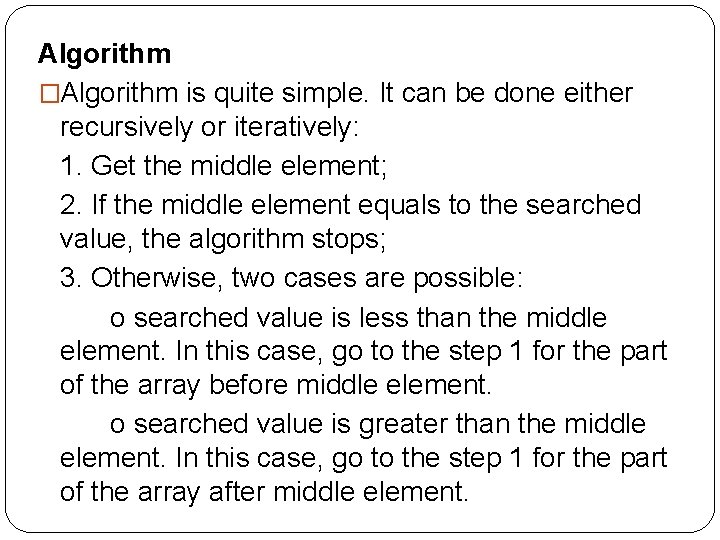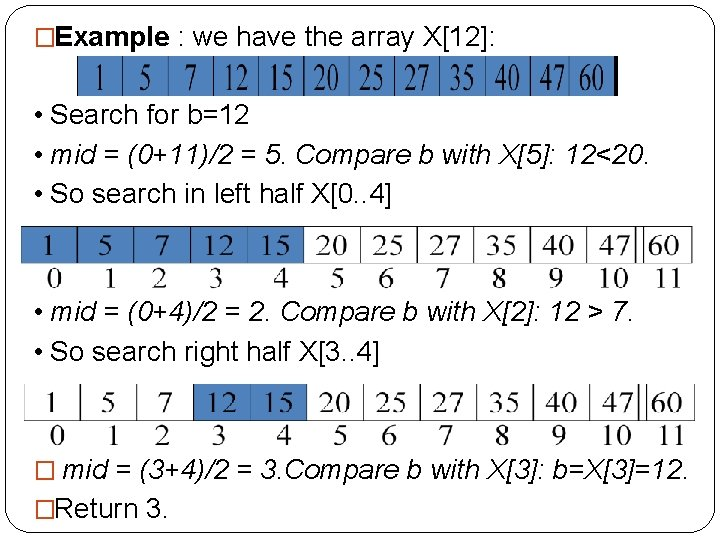# Searching Linear Sequential Search array or list by

• Slides: 7
Download presentationSearchingLinear (Sequential) Search array or list by checking items one at a time. �Search an �Linear search is usually very simple to implement, and is practical when the list has only a few elements, or when performing a single search in an unordered list. � Look at every element : This is a very straightforward loop comparing every element in the array with the key. As soon as an equal value is found, it returns. If the loop finishes withoutint Linear. Search(const int *Array, const int Size, const int Val. To. Search) { bool Not. Found = true; int i = 0; while(i < Size && Not. Found) { if(Val. To. Search != Array[i]) i++; else Not. Found = false; } if( Not. Found == false ) return i; else return -1; }Binary search algorithm � Search a sorted array by repeatedly dividing the search interval in half. �Begin with an interval covering the whole array. If the value of the search key is less than the item in the middle of the interval, narrow the interval to the lower half. Otherwise narrow it to the upper half. Repeatedly check until the value is found or the interval is empty. � A fast way to search a sorted array is to use a binary search. The idea is to look at the element in the middle. If the key is equal to that, the search is finished. If the key is less than the middle element,Algorithm �Algorithm is quite simple. It can be done either recursively or iteratively: 1. Get the middle element; 2. If the middle element equals to the searched value, the algorithm stops; 3. Otherwise, two cases are possible: o searched value is less than the middle element. In this case, go to the step 1 for the part of the array before middle element. o searched value is greater than the middle element. In this case, go to the step 1 for the part of the array after middle element.�Example : we have the array X: • Search for b=12 • mid = (0+11)/2 = 5. Compare b with X: 12<20. • So search in left half X[0. . 4] • mid = (0+4)/2 = 2. Compare b with X: 12 > 7. • So search right half X[3. . 4] � mid = (3+4)/2 = 3. Compare b with X: b=X=12. �Return 3.